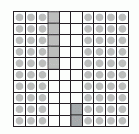Name: ___________________Date:___________________

 Email us to get an instant 20% discount on highly effective K-12 Math & English kwizNET Programs!

 Write the decimals one below the other. Note: The decimal point must be aligned and the numbers one below the other. Example: Add 0.35 and 0.42 Consider a square being divided into 100 equal parts.Shade 3 tenths and colour five hundreths which shows 0.35. Shade 4 tenths and colour 2 hundreths which shows 0.42. Count the total number of tenths and hundreths in the square.Answer: 0.77 Directions: Add the following decimal numbers. Also write at least 10 examples of your own.Name: ___________________Date:___________________

 Q 1: 56.31 + 4.2 =Answer: Q 2: 13.22 + 2.88 =Answer: Q 3: 42 + 8.994 =Answer: Q 4: 24.3 + 2.814 =Answer: Q 5: 16.3 + 21.55 =Answer: Q 6: 11.1 + 22.22 =Answer: Q 7: 29 + 8.443 =Answer: Q 8: 100 + .056 =Answer: Question 9: This question is available to subscribers only! Question 10: This question is available to subscribers only!